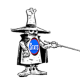BUS 233### Technology vs Tables and Rounding Mistakes

Joe, Two important points you bring up: Do not round intermediate values in a string of calculations. Wait until the very last step/answer to round to the number of decimals required by MyStatLab. On this problem and many others in this course, rounding too early can lead to wrong answers. In the instructor view, I

### Chebychev’s Theorem Excel Calculator

Because it is not often used, save in intro stats courses, and is relatively simple to calculate, most stats software programs (StatCrunch included) do not have a special tool to help. Instead, you will need to manually recreate the formula using Excel or a calculator. To make the process easier, I have built an Excel

### Do I need to know the Normal Approximation?

Section 5.5: Normal Approximation to the Binomial Distribution The premise in our Larson textbook for using the normal approximation is that finding the binomial probability of an “less than x” or “greater than x” problem would be onerous – having to manually calculate each of the discrete values and then sum them up. They give

### A bigger hoop is better: why confidence intervals get wider as the confidence increases

Joe, I think you are understanding confidence intervals, but I am not sure I am understanding your comments. So, let me restate them a bit. A 95% confidence interval means that if you repeat exactly the same process/experiment many times, 95% of the time the population parameter, e.g. the mean, will fall within that interval.

### A bigger hoop is better: why confidence intervals get wider as the confidence increases-2